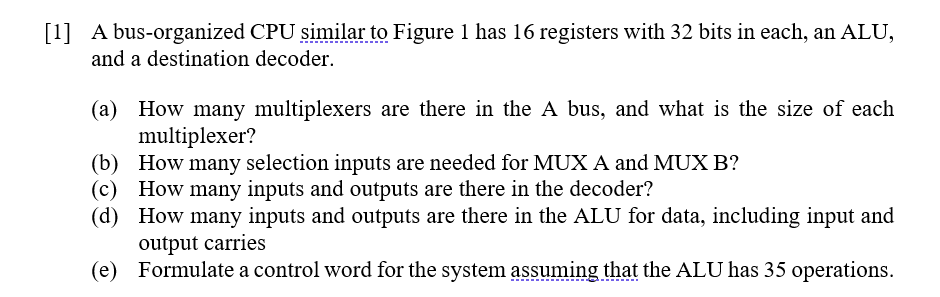# Question Computer Architecture: I want the text solution  A bus-organized CPU similar to Figure 1 has 16 registers with 32 bits in each, an ALU, and a destination decoder. (a) How many multiplexers are there in the A bus, and what is the size of each multiplexer? (b) How many selection inputs are needed for MUX A and MUX B? (C) How many inputs and outputs are there in the decoder? (d) How many inputs and outputs are there in the ALU for data, including input and output carries (e) Formulate a control word for the system assuming that the ALU has 35 operations.DMES2W The Asker · Computer Science
Computer Architecture: I want the text solutionTranscribed Image Text:  A bus-organized CPU similar to Figure 1 has 16 registers with 32 bits in each, an ALU, and a destination decoder. (a) How many multiplexers are there in the A bus, and what is the size of each multiplexer? (b) How many selection inputs are needed for MUX A and MUX B? (C) How many inputs and outputs are there in the decoder? (d) How many inputs and outputs are there in the ALU for data, including input and output carries (e) Formulate a control word for the system assuming that the ALU has 35 operations.
More
Transcribed Image Text:  A bus-organized CPU similar to Figure 1 has 16 registers with 32 bits in each, an ALU, and a destination decoder. (a) How many multiplexers are there in the A bus, and what is the size of each multiplexer? (b) How many selection inputs are needed for MUX A and MUX B? (C) How many inputs and outputs are there in the decoder? (d) How many inputs and outputs are there in the ALU for data, including input and output carries (e) Formulate a control word for the system assuming that the ALU has 35 operations.